﻿ Reliability Level of Smelting Gross Energy Requirement (SGER) Dependence on Direct Fuel Input and Process Free Energy Change

### Reliability Level of Smelting Gross Energy Requirement (SGER) Dependence on Direct Fuel Input and Pr...

C. I. Nwoye, M. O. Nwankwo, E. M. Ameh, N. E. Idenyi, L. C. Oshionwu, S. A. AbellaOPEN ACCESSPEER-REVIEWED

## Reliability Level of Smelting Gross Energy Requirement (SGER) Dependence on Direct Fuel Input and Process Free Energy Change

C. I. Nwoye1,, M. O. Nwankwo2, E. M. Ameh3, N. E. Idenyi2, L. C. Oshionwu2, S. A. Abella4

1Department of Metallurgical and Materials Engineering, NnamdiAzikiwe University, Awka, Nigeria

2Department of Industrial Physics, Ebonyi State University, Abakiliki, Nigeria

3Department of Metallurgical and Materials Engineering, Enugu State University of Science & Technology, Enugu, Nigeria

4Metallurgical Training Institute, Onitsha, Nigeria

### Abstract

This paper presents an assessment of the reliability level of Smelting Gross Energy Requirement (SGER) dependence on the direct fuel input and free energy change during industrial processing of engineering materials and minerals. A two-factorial polynomial-logarithmic model was derived and validated for empirical analysis of the dependent-independent variable relationship, which invariably aided reliability level evaluation. The validity of the model; ζ = - 0.071 ϑ 3 + 1.9885 ϑ 2 + 9.3181 ϑ - 0.0035 ɤ + 0.006 ln ɤ + 15.586 was rooted on the core model expression ζ - 15.586 = - 0.071ϑ 3 + 1.9885ϑ2 + 9.3181ϑ - 0.0035 ɤ + 0.006 ln ɤ where both sides of the expression were correspondingly nearly equal. The derived model was used to generate results of SGER, and its trend of distribution was compared with that from experimental results as a means of verifying its validity. The results of this verification translated into very close alignment of curves and significantly similar trend of data point’s distribution for experimental and derived model-predicted results. Evaluations from generated results indicated that SGER per unit direct fuel input & free energy change as obtained from experiment and derived model were 8.9260 and 9.0471 & 15.7474 and 15.9609 respectively. The evaluated measure of variability in experimental and model-predicted data sets relative to direct fuel input and free energy change were 121.6667 and 119.7662 as well as 123.1929 and 120.6838 respectively. Deviational analysis indicated that the maximum deviation of model-predicted SGER from the experimental results was less than 9.6%. This translated into over 90% operational confidence and reliability level for the derived model as well as 0.9 reliability coefficient for the SGER dependence on direct fuel input and free energy change accompanying the smelting process.

### At a glance: Figures

1234
Prev Next

• Nwoye, C. I., et al. "Reliability Level of Smelting Gross Energy Requirement (SGER) Dependence on Direct Fuel Input and Process Free Energy Change." American Journal of Industrial Engineering 2.2 (2014): 21-28.
• Nwoye, C. I. , Nwankwo, M. O. , Ameh, E. M. , Idenyi, N. E. , Oshionwu, L. C. , & Abella, S. A. (2014). Reliability Level of Smelting Gross Energy Requirement (SGER) Dependence on Direct Fuel Input and Process Free Energy Change. American Journal of Industrial Engineering, 2(2), 21-28.
• Nwoye, C. I., M. O. Nwankwo, E. M. Ameh, N. E. Idenyi, L. C. Oshionwu, and S. A. Abella. "Reliability Level of Smelting Gross Energy Requirement (SGER) Dependence on Direct Fuel Input and Process Free Energy Change." American Journal of Industrial Engineering 2, no. 2 (2014): 21-28.

 Import into BibTeX Import into EndNote Import into RefMan Import into RefWorks

### 1. Introduction

The high cost of useful energy for domestic use, research & development of more significantly reactive materials, better operational techniques and approach as well as industrial growth has necessitated consideration of energy analysis prior to materials and mineral processing in the industries. It sets up a bridge between engineering and economic analysis. The essence of an energy analysis is to evaluate the total quantity of energy (energy content of the fuels used) that has to be sequestrated from primary energy resources in order to produce a specified commodity .

The total energy content of the primary fuel inputs denoted by Ein has been reported  to equal the sum of the quantities of fuel, xf, multiply by their respective energy contents, Ef. Furthermore, the total ger of the outputs equals the sum of the quantities of each commodity, xi, multiply by its respective ger, Ei. Therefore, for the whole economy, as shown in Figure 1;

Figure 1. Relationship between industrial economy, primary fuel inputs and commodity outputs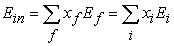(1)

The aim of energy analysis, which is to attribute all the primary fuels to the final outputs, implies that in the industrial economy, there cannot be any loss of energy, though in practice energy are obviously lost perhaps as waste heat.

Energy requirement is basically a technical measure common to all processes. Furthermore, theoretical energy requirement is a measure of draw back in bringing about expected transformations in the process. A process with larger energy requirement is more difficult to accomplish. Energy requirement could therefore be used as an index of the technical cost of accomplishing the required transformation. In order to incorporate the technical efficiency and the basic possibility of technical improvements, it is necessary to make use of the actual fuel used, rather than the theoretical energy requirement, as the index of costs, though the actual fuel used in smelting depends on both the theoretical energy required, calculated from the smelting reactions used and upon the efficiency with which fuel is used in furnaces. Based on the foregoing, the quantity of fuel used in a process is taken as a good proxy of the technical cost of production.

The direct fuel was simply reported  as the fuel used in the smelting stage and does not include any over head for conversion nor does it include any other materials used in the process.

Research  has revealed three broad classes of processes associating the production of metals from ores. In the first class of the process, energy is provided to separate the metal from its mineral. This is fundamentally a chemical process limited by the change in Gibbs free energy associated with the change in chemical composition. The second class of processes is that concerned with sorting or changing concentrations. In theory, such processes are governed by the associated change in entropy; in practice, other factors dominate. The third class of processes is transport. This lies outside classical thermodynamics which is significantly concerned with equilibrium states and infinitely slow changes from one state to another.

Fuel use in the production of goods and services were analyzed, basically in relation to the study of food production system [3, 4], housing , resource availability  and the production and recycling of metals .

The analysis includes the direct use of fuels in the production process and also the fuel used to produce materials and machines in the process.

The result of the analysis is the gross energy requirement of the commodity, or ger. The sequestrated energy does not include energy extracted from the environment or from non-fuel materials such sulphur.

Imports are included in the energy analyses of economies in several ways: (1) counting the import as having the same ger as the equivalent home produced goods, (2) counting it on the basis of the ger incurred in country of manufacture, (3) considering solely the use of fuel within the economy and counting the imports with zero ger.

It has been reported  that comparison of different materials should be done on the basis of the specific material property exploited in application, which varies with gross energy requirement (GER) associating production of the material.

The aim of this work is to evaluate the reliability level of smelting gross energy requirement (SGER) dependence on the direct fuel input and free energy change during industrial processing of engineering materials and minerals. The model is expected to generate results that will be in proximate agreement with experimental results.

### 2. Materials and Methods

Ores of corresponding metals such as lead, copper, zinc, titanium, magnesium, and aluminium were smelted following conventional step-wise processes. Details of the experimental procedure and associated process conditions are as stated in the past report [8, 9, 10, 11].

2.1. Model Formulation

Experimental data obtained from research work [8, 9, 10, 11] were used for this work. Computational analysis of these data shown in Table 1, gave rise to Table 2 which indicate that;(2)

Introducing the values of Se, S, K, N, Ne, and Ke into equation (2) reduces it to;(3)(4)

Where

(ϑ) = Free energy change (MJ/kg)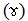= Direct fuel input (MJ/kg)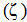= SGER (MJ/kg)

Se = 15.586, S = 0.071, K = 1.9885,N = 9.3181, Ne = 0.0035 and Ke = 0.006. Se, S, K, N, Ne, and Ke are empirical constants (determined using C-NIKBRAN .

Equation (4) is the derived model and is referred to as Smelting Gross Energy Requirement Model. (SGER Model).

### 3. Boundary and Initial Conditions

Consider smelting of various ores from which corresponding metals such as lead, copper, zinc, titanium, magnesium, and aluminium were produced. Range of SGERs, free energy change and direct fuel input, considered were: 19 - 430MJ/kg, 0.45 - 29.2 MJ/kg and 8.1-177 MJ/kg respectively. The derived model is applicable within the scope of producing lead, copper, zinc, titanium, magnesium, and aluminium through smelting of their respective ores.

### 4. Results and Discussion

The derived model (SGER) is equation (4). Computational analysis of Table 1 gave rise to Table 2

#### Table 2. Variation of ζ - 15.586 with - 0.071ϑ3 + 1.9885ϑ2 + 9.3181ϑ- 0.0035ɤ + 0.006 ln ɤ

4.1. Model Validation

The validity of the model was found to be rooted on the core model expression (equation (3)) where both sides of the equation were correspondingly nearly equal. The differences were due to deviations of model-predicted SGERs from the corresponding experimental results. Table 2 also agrees with equation (3) following the values of ζ - 15.586 and - 0.071ϑ 3 + 1.9885ϑ2 + 9.3181ϑ - 0.0035ɤ + 0.006lnɤ precisely evaluated from the experimental results in Table 1. In addition, the derived model was validated by comparing the SGER predicted by the model and that obtained from the experiment [8, 9, 10, 11]. This was done using the 4th Degree Model Validity Test Techniques (4th DMVTT); computational, graphical, statistical and deviational analysis .

Figure 4. Coefficient of determination between SGER and process free energy change as obtained from experiment [8,9,10,11]
Figure 6. Comparison of SGERs (relative to direct fuel input) as obtained from experiment [8,9,10,11] and derived model (line distribution curve)
Figure 7. Comparison of SGERs (relative to direct fuel input) as obtained from experiment [8,9,10,11] and derived model (trend curve)
Figure 8. Comparison of SGERs (relative to process free energy change) as obtained from experiment [8,9,10,11] and derived model
4.2. Statistical Analysis
4.2.1. Measure of Variability (AVEDEV)

The measure of variability in experimental and model-predicted data sets relative to direct fuel input and free energy change were 121.6667 and 119.7662 as well as 123.1929 and 120.6838 respectively. The standard error was evaluated using Microsoft Excel version 2003.

4.2.2. Correlation (CORREL)

The correlation coefficient between SGER and direct fuel input & process free energy change were evaluated from the results of derived model and experiment in Figure 2 - Figure 5, considering the coefficient of determination R2. This was calculated using Microsoft Excel version 2003.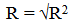(5)

The evaluated correlations are shown in Table 3. These evaluated results indicate that the derived model predictions are significantly reliable and hence valid considering its proximate agreement with results from actual experiment.

#### Table 4. Comparison of the correlations evaluated from derived model predicted and ExD results based on process free energy change

4.3. Graphical Analysis

Comparative graphical analysis of Figure 6 and Figure 7 shows very close alignment of curves and significantly similar trend of data point’s distribution for experimental and model-predicted results of SGER. This shows proximate agreement between experimental and derived model-predicted results.

4.4. Computational Analysis

Comparative computational analysis of the experimental and model-predicted SGER was carried out to ascertain the degree of validity of the derived model. This was done by comparing results of evaluated SGERs per unit direct fuel input and per unit process free energy change as obtained from experimental and derived model within direct fuel input and process free energy change range: 8.1-177 MJ/kg and 19 – 430 MJ/kg respectively.

4.4.1. SGER Per Unit Process Free Energy Change

SGER per unit process free energy change ζ / ϑ was calculated from the equation;(6)

Re-written as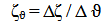(7)

Equation (7) is detailed as(8)

Where

Δζ = Change in the SGERs ζ2, ζ 1 at two values of the process free energy change ϑ 2 - ϑ 1.

Considering the points (0.45, 19) & (24.2, 393) and (0.45, 20.1595) & (24.2, 399.231) as shown in Figure 6, and designating them as (ζ1, ϑ1) & (ζ2 ϑ2) for experimental and derived model predicted results respectively, and then substituting them into equation (8), gives the slopes: 15.7474 and 15.9609 respectively as their corresponding SGER per unit process free energy change.

4.4.2. SGER Per Unit Direct Fuel Input

Similarly, substituting into equation (8) points (8.1, 19) & (50, 393) and (8.1, 20.1595) & (50, 399.231) as shown in Figure 7, and designating them as (ζ1, ɤ1) & (ζ2, ɤ2) for experimental and derived model predicted results respectively also evaluates the slopes: 8.9260 and 9.0471 respectively as their corresponding SGER per unit direct fuel input. The proximity between values in each result set indicates significantly high validity level for the derived model.

4.5. Deviational Analysis

Comparative analysis of SGERs obtained from experiment and derived model shows insignificant deviations on the part of the model-predicted values relative to values obtained from the experiment. This is attributed to the fact that the surface properties of the ore and the physiochemical interactions between the ore and added reducing agent, which played vital roles during the smelting process (affecting the process free energy change) were not considered during the model formulation. This viably necessitated the introduction of correction factor, to bring the model-predicted SGER to those of the corresponding experimental values.

The deviation Dv, of model-predicted SGER from the corresponding experimental result is given by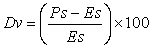(9)

Correction factor, Cf to the model-predicted results is given by(10)

Where

Es and Ps are SGERs evaluated from experiment and derived model respectively.

Deviational analysis in Figure 9 and Figure 10 indicate that the precise maximum deviation of model-predicted SGER from the experimental results is less than 9.6%. This translates into over 90% operational confidence and reliability level for the derived models and well over 0.9 reliability coefficient for the SGER dependence on direct fuel input and process free energy change.

Figure 10. Variation of deviation with process free energy change (relative to the direct fuel use)

Consideration of equation (9) and critical analysis of Figure 2-Figure 5, Figure 9 and Figure 10 show that the least and highest magnitudes of deviation of the model-predicted SGER (from the corresponding experimental values) were + 1.59 and + 9.5%. This corresponds to SGERs: 399.231 and 60.226 MJ/kg, direct fuel inputs: 50 and 25.3 MJ/kg as well as process free energy changes: 24.2 and 3.04 MJ/kg respectively.

Equations (9) and (10) indicate that correction factor is the negative of the deviation.

The correction factor took care of negligence of the operational contributions of surface properties of the ore being smelted and the physiochemical interactions between the ore and added reducing agent which really affected the free energy change during the smelting process. The model predicted results deviated from those of the experiment because these contributions were not considered during the model formulation. Introduction of the corresponding values of Cf from equation (10) into the model gives exactly the corresponding experimental SGER.

#### Table 5. Variation correction factor (to model-predicted SGER) with direct fuel input and process free energy change

Comparative analysis of equation (10) and Table 5 indicates that the evaluated correction factors are negative of the deviation as shown in equations (9) and (10). Further comparative analysis Figure 2-Figure 5, Figure 9 and Figure 10 show that the least and highest correction factor (to the model-predicted SGER) were - 1.59 and - 9.5% which correspond to SGERs: 399.231 and 60.226 MJ/kg, direct fuel inputs: 50 and 25.3 MJ/kg as well as process free energy changes: 24.2 and 3.04 MJ/kg respectively.

It is important to state that the deviation of model predicted results from that of the experiment is just the magnitude of the value. The associated sign preceding the value signifies that the deviation is a deficit (negative sign) or surplus (positive sign).

### 5. Conclusion

An assessment of the reliability level of SGER dependence on the direct fuel input and free energy change during industrial processing of engineering materials and minerals has been carried out. A two-factorial polynomial-logarithmic model derived, validated and used for empirical analysis of the dependent-independent variable relationship, significantly aided the reliability level evaluation. The validity of the model was rooted on the core model expression ζ - 15.586 = - 0.071ϑ 3 + 1.9885ϑ2 + 9.3181ϑ - 0.0035 ɤ + 0.006 ln ɤ where both sides of the expression are approximately equal. Evaluations from generated results indicated that SGER per unit direct fuel input and free energy change as obtained from experiment and derived model were 8.9260 and 9.0471 and 15.7474 and 15.9609 respectively. The evaluated measure of variability in experimental and model-predicted data sets relative to direct fuel input and free energy change were 121.6667 and 119.7662 as well as 123.1929 and 120.6838 respectively. Deviational analysis indicated that the maximum deviation of model-predicted SGER from the experimental results was less than 9.6%. This translated into over 90% operational confidence and reliability level for the derived model as well as 0.9 reliability coefficient for the SGER dependence on direct fuel input and free energy change accompanying the smelting process.

### References

  Chapman, P. F., and Roberts, F. (1983). Metal Resources and Energy, Butterworths, London, pp. 96-120.In article CrossRef  Alexander, W. O. (1979). Total Energy Content and Costs of Some Significant Materials in Relation to their Properties and Availability, in Evaluation of Energy Use, The Watt Committee on Energy Report No. 6, The Watt Committee London.In article  Leach, G. (1973). The Energy Cost of Food Production in The Man-Food Equation, ed. A. Bourne, Academic Press.In article  Slesser, M. (1973). Energy Subsidy as a Criterion Food Policy Planning` Journal of Science of Food and Agriculture, 24.In article  MacKillop, A. (1972). `Low energy Housing`, Ecologist, 2:12.In article  Chapman, P. F. (1973). No Overdrafts in the Energy Economy, New Scientist, 58:408.In article  Bravard, J. C., Flora, H. B., and Portal, C. (1972). `Energy Expenditure Associated with the Production and Recycle of Metals`.ORNL-NSF-EP 24, Oak Ridge Nat. Lab.In article  Energy Use Patterns in Metallurgical and Non-Metallic Mineral Processing, Batelle-Columbus Laboratories, US Bureau of Mines OFR 80-75, 1975.In article  Chapman, P. F. (1973). The Energy Costs of Producing Copper and Aluminium from Primary Sources, Research Report ERG001, Open University.In article  Kellog, H. H. (1976). Sizing Up the Energy Requirements for Producing Primary Materials, Engineering and Mining Journal, 178(4):29.In article  Philips, W. G. B and Edwards, D. P. (1976). Metal Prices as a Function of Ore Grade, Resources Policy, pp. 167.In article CrossRef  Nwoye, C. I. (2008). C-NIKBRAN-Data Analytical Memory (Software).In article  Nwoye, C. I., and Nwabanne J. T. (2013). Empirical Analysis of Methane Gas Yield Dependence on Organic Loading Rate during Microbial Treatment of Fruit Wastes in Digester. Advances in Applied Science Research 4(1):308-318.In article